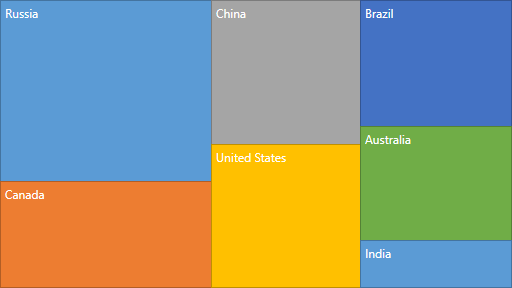# SquarifiedLayoutAlgorithm Class

A class representing the Squarified layout algorithm.

Namespace: DevExpress.Xpf.TreeMap

Assembly: DevExpress.Xpf.TreeMap.v22.2.dll

NuGet Package: DevExpress.Wpf.TreeMap

## Declaration

``````public class SquarifiedLayoutAlgorithm :
TreeMapLayoutAlgorithm``````

## Remarks

The idea of the algorithm is to layout the rectangles along the required direction in the available display area as long as the highest width/height ratio of any rectangle in the current row keeps improving. Once the highest aspect ratio among the rectangles has reached a minimum, and adding another rectangle to the current row would increase the worst aspect ratio, the current row is fixed and a new row is created.

The following image demonstrates the tree map layout calculated using this algorithm.## Example

To customize the layout algorithm, do the following.

``````<dxtm:TreeMapControl x:Name="treeMap"
LayoutAlgorithm="{Binding ElementName=lbLayoutAlgorithm, Path=SelectedValue}">
<!-- endregion #LayoutAlgorithm-->
<dxtm:TreeMapControl.Colorizer>
<dxtm:TreeMapPaletteColorizer/>
</dxtm:TreeMapControl.Colorizer>
<dxtm:TreeMapItemStorage>
<dxtm:TreeMapItem Label="Americas">
<dxtm:TreeMapItem Label="United States" Value="17.418"/>
<dxtm:TreeMapItem Label="Brazil" Value="2.353"/>
</dxtm:TreeMapItem>
<dxtm:TreeMapItem Label="Europe">
<dxtm:TreeMapItem Label="Germany" Value="3.859"/>
<dxtm:TreeMapItem Label="United Kingdom" Value="2.945"/>
<dxtm:TreeMapItem Label="France" Value="2.846"/>
<dxtm:TreeMapItem Label="Italy" Value="2.147"/>
<dxtm:TreeMapItem Label="Russia" Value="1.857"/>
</dxtm:TreeMapItem>
<dxtm:TreeMapItem Label="Asia">
<dxtm:TreeMapItem Label="China" Value="10.380"/>
<dxtm:TreeMapItem Label="Japan" Value="4.616"/>
<dxtm:TreeMapItem Label="India" Value="2.049"/>
</dxtm:TreeMapItem>
</dxtm:TreeMapItemStorage>
<!--region #CloseTag-->
</dxtm:TreeMapControl>
<!--endregion #CloseTag-->
<Grid Grid.Column="1" Margin="4">
<Grid.RowDefinitions>
<RowDefinition Height="Auto"/>
<RowDefinition Height="Auto"/>
<RowDefinition Height="Auto"/>
<RowDefinition Height="Auto"/>
</Grid.RowDefinitions>
<TextBlock Grid.Row="0"
Margin="0,0,0,4"
Text="Layout Algorithm:"/>
<!--region #LayoutAlgorithmList-->
<ListBox x:Name="lbLayoutAlgorithm"
Grid.Row="1"
DisplayMemberPath="LayoutAlgorithmName"
SelectedValuePath="LayoutAlgorithm"
SelectedIndex="0">
<local:LayoutAlgorithmWarapper LayoutAlgorithmName="Slice and Dice">
<dxtm:SliceAndDiceLayoutAlgorithm/>
</local:LayoutAlgorithmWarapper>
<local:LayoutAlgorithmWarapper LayoutAlgorithmName="Squarified">
<dxtm:SquarifiedLayoutAlgorithm/>
</local:LayoutAlgorithmWarapper>
<local:LayoutAlgorithmWarapper LayoutAlgorithmName="Striped">
<dxtm:StripedLayoutAlgorithm/>
</local:LayoutAlgorithmWarapper>
</ListBox>
``````

The following code snippet (auto-collected from DevExpress Examples) contains a reference to the SquarifiedLayoutAlgorithm class.

Note

The algorithm used to collect these code examples remains a work in progress. Accordingly, the links and snippets below may produce inaccurate results. If you encounter an issue with code examples below, please use the feedback form on this page to report the issue.